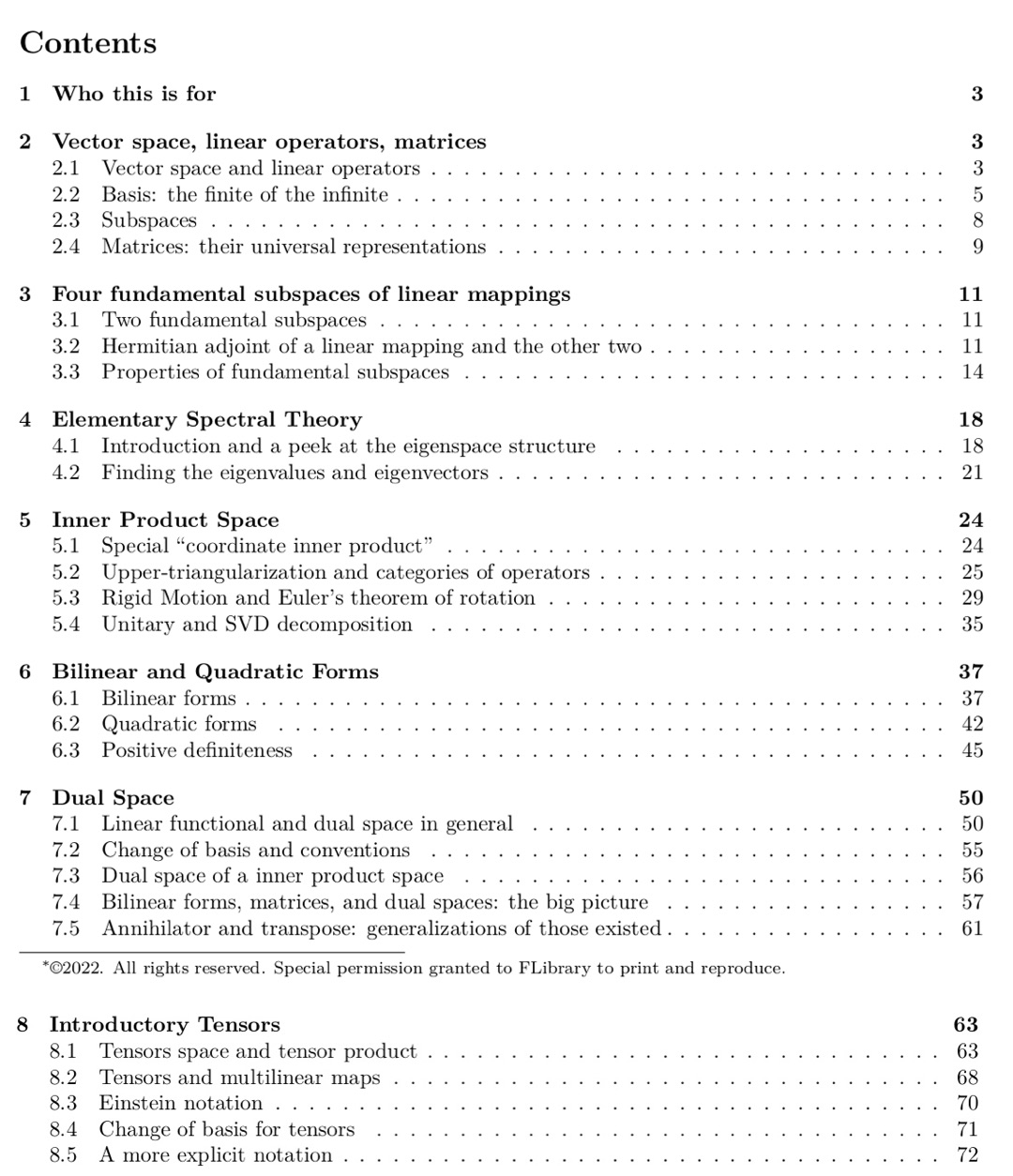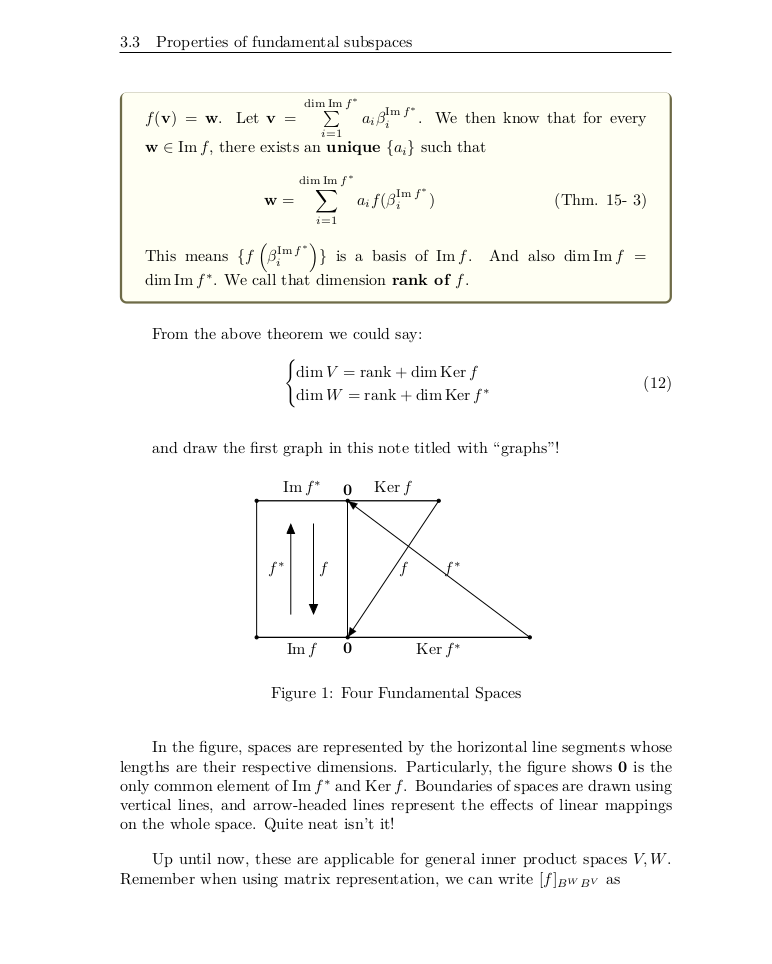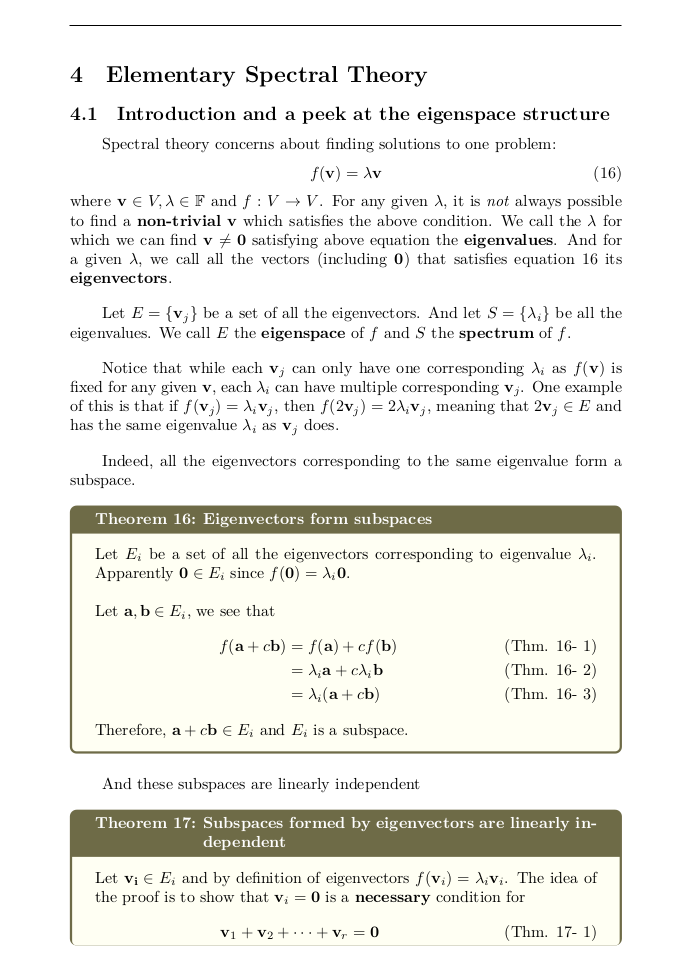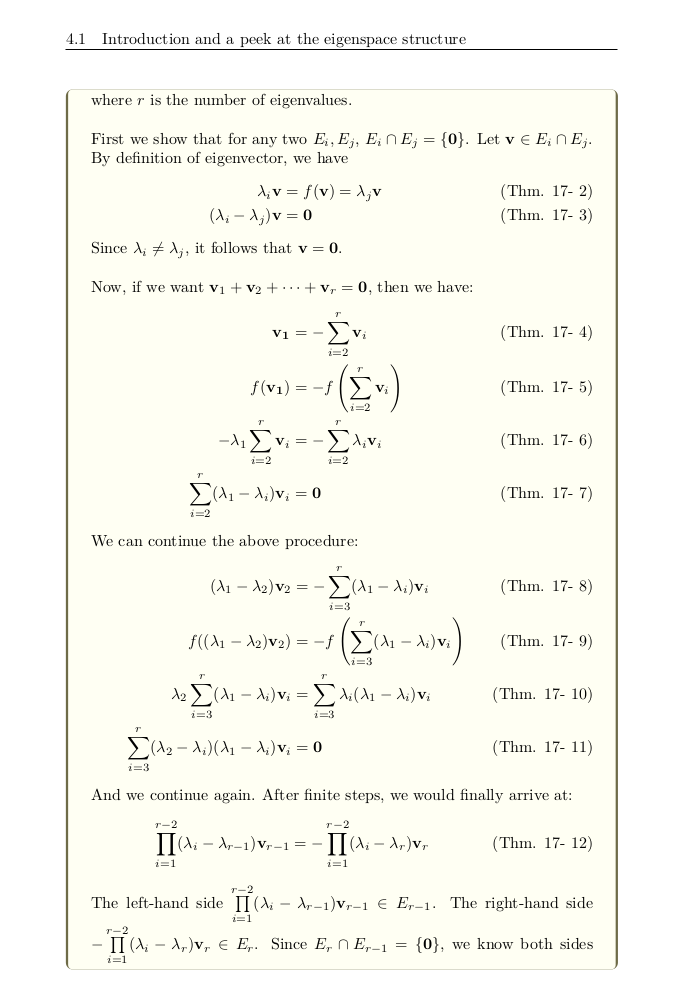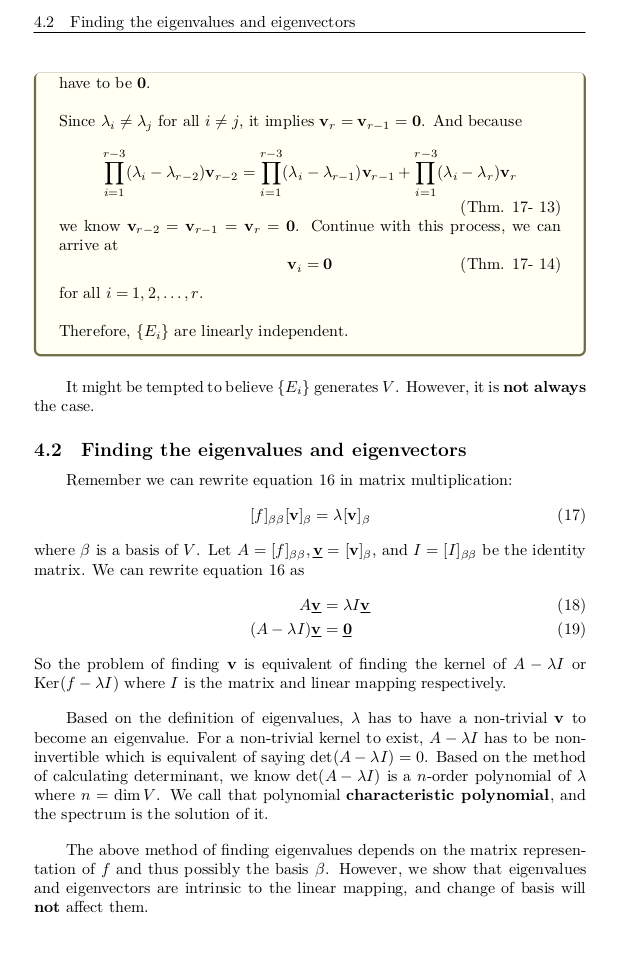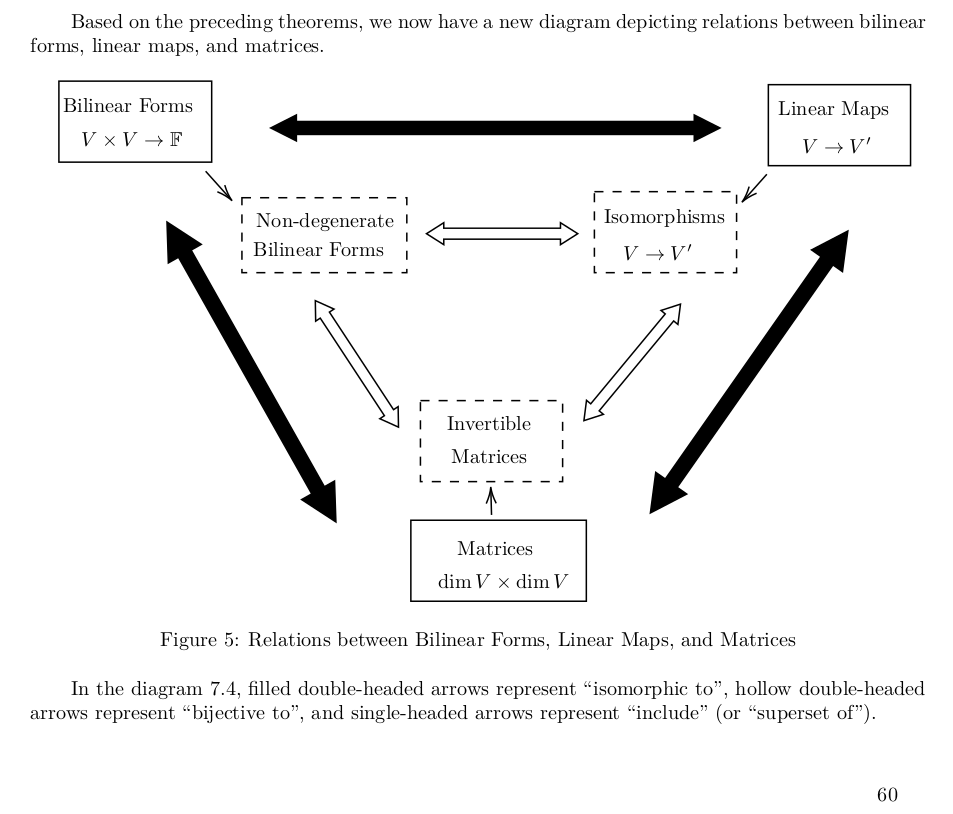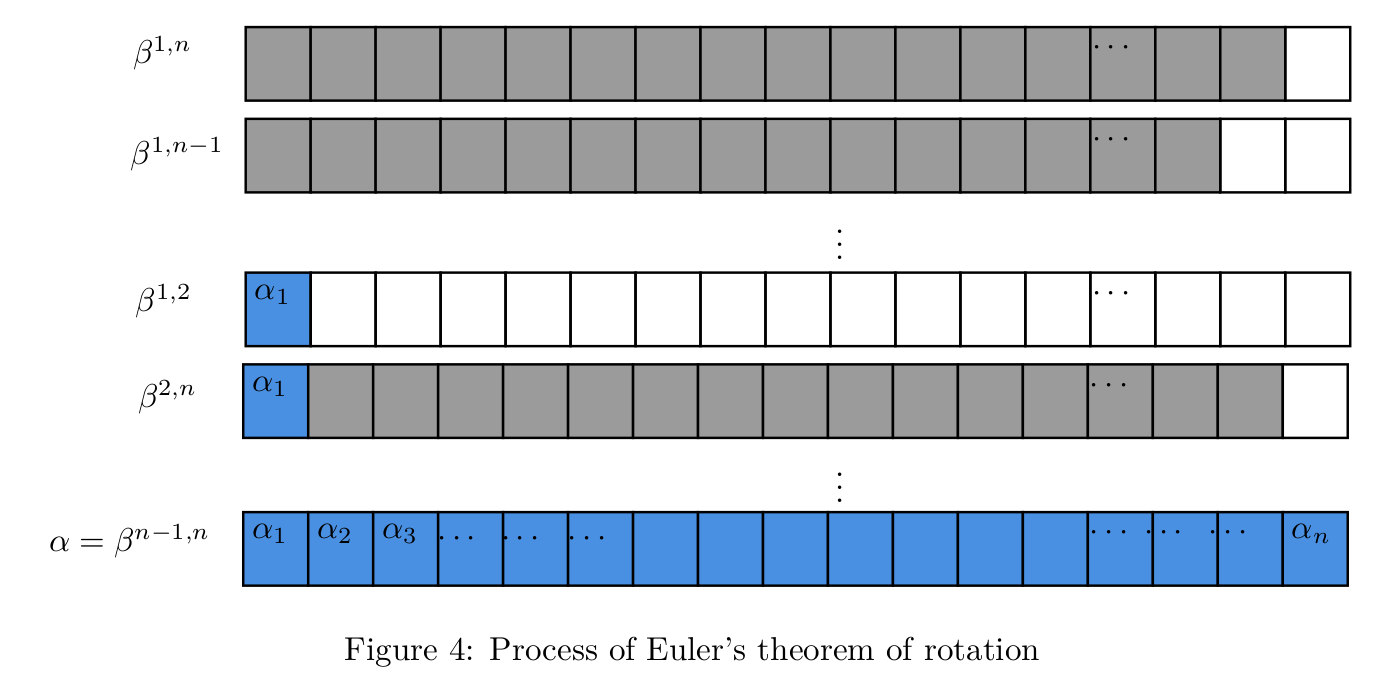# Linear Algebra in Diagrams

ID 3925724387 USD 0 9969 数学 | Mathematics Verified

Linear Algebra in Diagrams is a linear algebra notebook based on Linear Algebra Done Wrong, featuring:

• Diagrams Are used as much as possible to visualize theorems and proofs and summarize chapters, building intuition for you.
• Coherent and concise introduction to almost everything from linear spaces to tensors.
• Attempt to explain the motivation behind the construction of concepts, symbols, and theorems.

It is suitable as:

• A supplement for beginners in linear algebra.
• A quick review handbook for the proofs and concepts
• A tool to improve intuitive understanding of some fundamental theorems

The notes are currently in its first draft stage and may have errors such as typos. If you have any questions, you can contact the author, thank you very much.

Linear Algebra in Diagrams 是一份基于 Linear Algebra Done Wrong 的线性代数笔记，它的特点有：

• 尽可能通过图像具象化了定理的证明和结论，并总结章节内容，更好地来建立对它们的直觉。

• 连贯且精炼的介绍了从线性空间到张量初步的几乎所有内容。

• 尝试对概念、符号、定理构造的动机进行解释

• 作为初学线性代数时的补充

• 对基础定理的证明作快速复习

• 想提升对一些基础定理的直觉理解

笔记目前处于初稿阶段，可能存在 typo 等错误。如有疑问可以联系作者，感激不尽。